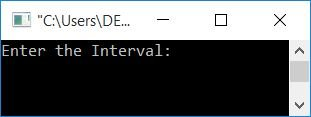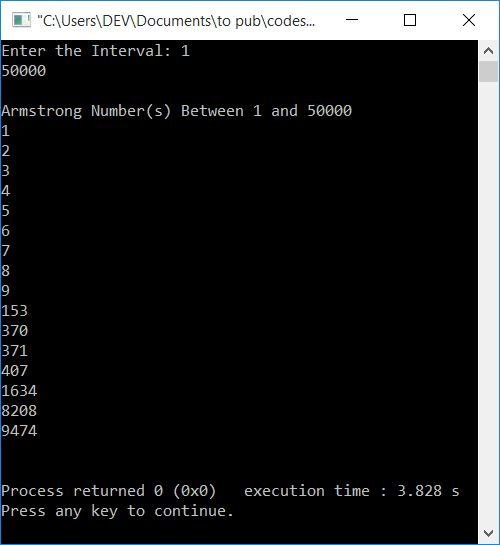# C++ Program to Generate Armstrong Numbers

In this article, you will learn and get code to generate and print Armstrong numbers in C++ programming. Here is the list of programs available here:

### What is an Armstrong number?

A number that equals the sum of its own digits, where each digit is raised to the power of the number of digits. For example, 8208 is an Armstrong number because:

```8208 = 84 + 24 + 04 + 84
= 4096 + 16 + 0 + 4096
= 4096 + 16 + 4096
= 8192 + 16
= 8208```

The result is equal to the number itself. So it is an Armstrong number.

Note: Because the total number of digits in 8208 is 4, each of its digits is raised to the power of 4.

## Print Armstrong numbers between 1 and 500

This program generates and prints all Armstrong numbers between 1 and 500.

The question is: write a program in C++ to generate and print Armstrong numbers between 1 and 500. Here is its answer:

```#include<iostream>
using namespace std;
int main()
{
int first, last, temp, noOfDigit, num, res=0, rem, pow, i;
first = 1;
last = 500;
cout<<"Armstrong Numbers Between 1 and 500\n";
while(first<=last)
{
temp = first;
noOfDigit = 0;
while(temp>0)
{
temp = temp/10;
noOfDigit++;
}
num = first;
while(num>0)
{
rem = num%10;
pow = 1;
i = 0;
while(i<noOfDigit)
{
pow = pow*rem;
i++;
}
res = res + pow;
num = num/10;
}
if(res==first)
cout<<res<<endl;
first++;
res = 0;
}
cout<<endl;
return 0;
}```

This program was built and runs under the Code::Blocks IDE. Here is its sample run:The program uses a while loop that starts with 1 and ends with 500. And each time the value of first is set to temperature. Now we've calculated the number of its digits. Then it checks whether the value of temp is an Armstrong number or not. If it is an Armstrong, then it prints its value as output; otherwise, the same process continues to check with the next number until the value of the first becomes greater than the last.

## Print the Armstrong number within the given range

To generate and print Armstrong numbers in a given range given by the user, using C++ programming, you have to ask the user to enter the interval. Then apply the same process as given in the previous program.

The only difference is that the program receives the value of first and last here.

```#include<iostream>
using namespace std;
int main()
{
int first, last, temp, noOfDigit, num, res=0, rem, pow, i;
cout<<"Enter the Interval: ";
cin>>first>>last;
cout<<"\nArmstrong Number(s) Between "<<first<<" and "<<last<<endl;
while(first<=last)
{
temp = first;
noOfDigit = 0;
while(temp>0)
{
temp = temp/10;
noOfDigit++;
}
num = first;
while(num>0)
{
rem = num%10;
pow = 1;
i = 0;
while(i<noOfDigit)
{
pow = pow*rem;
i++;
}
res = res + pow;
num = num/10;
}
if(res==first)
cout<<res<<endl;
first++;
res = 0;
}
cout<<endl;
return 0;
}```

Here is its sample run. This is the initial output produced by the above program:Now enter the interval, say 1 and 50000, to print all Armstrong numbers between 1 and 50000 as shown in the snapshot given below:#### The same program in different languages

C++ Quiz

« Previous Program Next Program »